# Tutorial¶

This tutorial will take you three the main steps of using `nbchkr`:

• Write an assignment with solutions and checks.
• Create a release notebook with the solutions and checks removed.
• For a collection of submissions: check the work and create individual feedback.

## Installing `nbchkr`¶

To install the latest release of `nbchkr`, at a command line interface run the following command:

```\$ python -m pip install nbchkr
```

## Writing an assignment¶

### Initial setup¶

Open a Jupyter notebook, we will choose to name it `main.ipynb` (but the name is not important).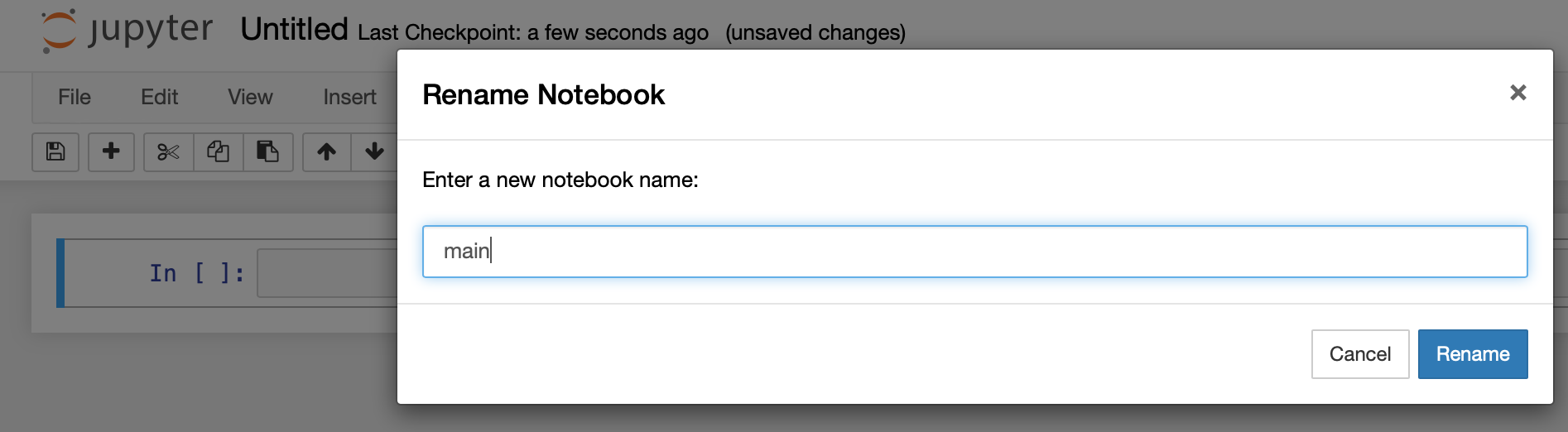On the Jupyter toolbar, click on `View` and then `Cell Toolbar` and then `Tags`.This should make the native tag menu available to you on every cell in your Jupyter notebook.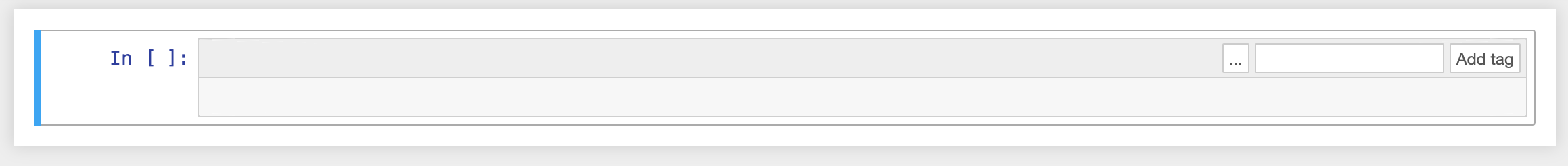We can now start writing our assignment.

### Writing text for a question¶

Let us write a markdown cell with some instructions and a question that we want to ask our students:

```# Class assignment

We will use this assignment to solidify our understanding of using Python to
carry out some numerical operations and also write functions.

Use this notebook to write your answers in the cells as instructed, do your
best not to delete any of the cells that are already there.

## Question one.

Use python to obtain the remainder when dividing 21 by 5.

\\[21 \mod 5\\]
```

Be sure to indicate that that cell is a `markdown` cell and not the usual `code` cell.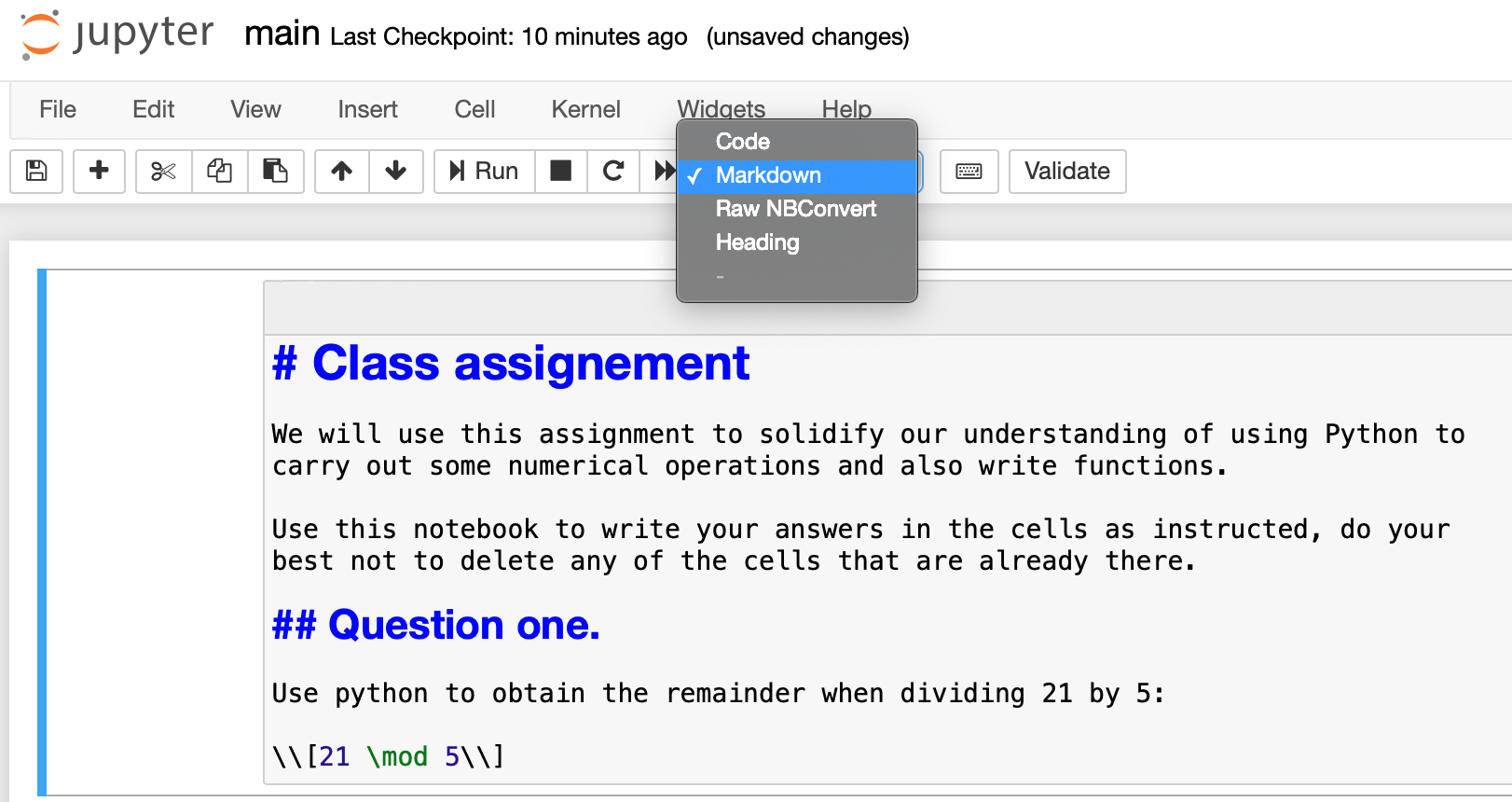Once you run that cell it should like like the following: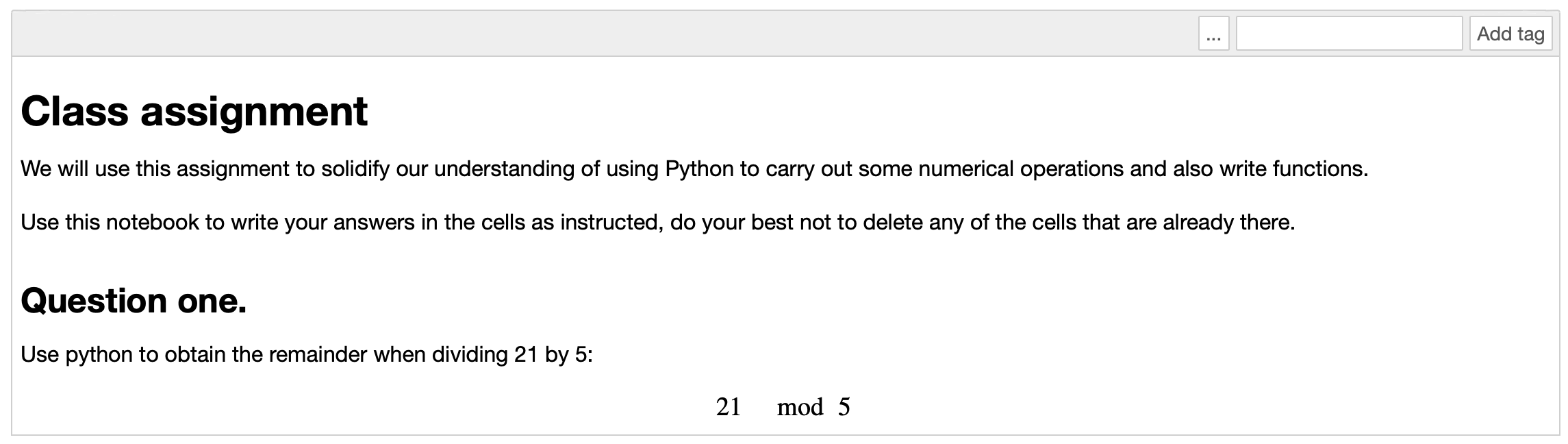### Writing the answer to a question¶

In the next cell we will write down the expected answer but also include a delimiters for what should not be shown to students:

```### BEGIN SOLUTION
21 % 5
### END SOLUTION
```

We can run that cell if we want to keep an eye on the answer.

An important step at this stage is to let `nbchkr` know that this is an answer cell, we do this by adding `answer:q1` to tags.

Everything should now look like the following: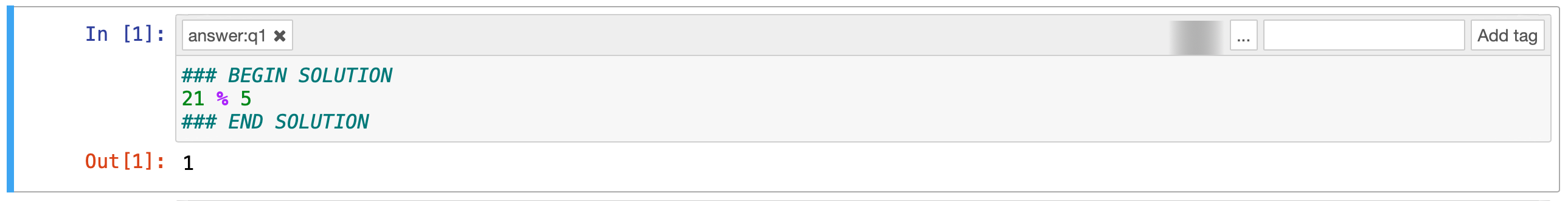### Writing checks for the answer¶

We will now write a check for the answer, that `nbchkr` uses to be able to give feedback to a student. We do this using python `assert` statements:

```q1_answer = _
feedback_text = "Your opteration did not return an integer which is expected"
assert type(q1_answer) is int
```

We will also add a tag: `score:1` to this cell.

As well as checking that the answer is an integer let us check the actual answer by creating a new cell and writing:

```feedback_text = "The expected answer is 1 because 21 = 5 * 3 + 1"
assert q1_answer == 1, feedback_text
```

This will be worth 3 points so let us add the tag: `score:3`.

We can choose to add a description to our check which will then appear in the feedback. We do this by adding the tag: `description:correct-answer`.

Everything should now look like the following:### Writing another question¶

Let us write a second question that asks students to write a function:

```## Question two.

Write a python function `get_remainder(m, n)` that returns the remainder
the remainder when dividing \\(m\\) by \\(n\\).

\\[m \mod n\\]
```

### Writing the answer¶

As before we write an answer in a cell below:

```def get_remainder(m, n):
### BEGIN SOLUTION
"""
This function returns the remainder of m when dividing by n
"""
return m % n
### END SOLUTION
```

### Including checks¶

We will now add some cells to check the answer.

First let us make sure there is a docstring:

```feedback_text = """You did not include a docstring. This is important to help document your code.

It is done  using triple quotation marks. For example:

def get_remainder(m, n):
\"\"\"
This function returns the remainder of m when dividing by n
\"\"\"
...

Using that it's possible to access the docstring,
one way to do this is to type: `get_remainder?`
(which only works in Jupyter) or help(get_remainder).

We can also comment code using `#` but this is completely
ignored by Python so cannot be accessed in the same way.

"""
assert  get_remainder.__doc__ is not None, feedback_text
```

Whilst we’ve decided to write quite a lot of feedback with details about writing docstrings we are only going to score this part of the answer 1 point so we use the tag: score:1.

We will add the description tag: `description:presence-of-docstring`.

We will also include specific checks for the actual answer:

```assert get_remainder(5, 3) == 2, "Incorrect answer for m=5, n=3: 5 mod 2 = 1 because 5 = 3 * 1 + 2"
assert get_remainder(34, 21) == 13, "Incorrect answer for m=34, n=21: 34 mod 21 = 13 because 34 = 21 * 1 + 13"
assert get_remainder(1000, 10) == 0, "Incorrect answer for m=1000, n=10: 1000 mod 10 = 0 because 1000 = 10 * 100 + 0"
```

For this we will use the description tag: `description:correct-answer`.

IF you would like to see a final version of this notebook you can find it `here`.

## Releasing an assignment¶

Now we can take that source notebook and create an assignment that can be given to students. To do this, we use the command line tool that comes with `nbchkr`:

```\$ nbchkr release --source main.ipynb --output assignment.ipynb
```

This creates `assignment.ipynb` with the answers and checks removed.

## Releasing solutions¶

If we want to create a model solution we can. To do this, we use the command line tool that comes with `nbchkr`:

```\$ nbchkr solve --source main.ipynb --output solution.ipynb
```

This creates `solution.ipynb` with the checks removed.

## Checking student assignments and generating feedback¶

Assuming we have a class of 3 students who each submitted a notebook with the following naming convention:

```assignment_<student_number>.ipynb
```

These notebooks are all put in a `submissions/` directory:

To check them and generate the feedback we again use the `nbchkr` command line tool:

```\$ nbchkr check --source main.ipynb --submitted "submissions/*.ipynb" --feedback-suffix -feedback.md --output data.csv
```

This has gone through and checked each notebook, you can see the output here:

The summary results
Submission filepath Score Maximum score Tags match
submissions/assignment_01.ipynb 2 11 True
submissions/assignment_02.ipynb 10 11 True
submissions/assignment_03.ipynb 4 11 False

We see that assignment_03.ipynb has a `False` flag under the `Tags Match` heading: this is because the student must have deleted one of the cells with a required tag. `nbchkr` does its best to check them anyway but this is a notebook that we should check manually.

In the submissions directory, 3 markdown files have been written with feedback to the students:

`assignment_01.ipynb-feedback.md`:

```
---

1 / 1

The expected answer is 1 because 21 = 5 * 3 + 1

0 / 3

---

### Presence of docstring

1 / 1

Incorrect answer for m=5, n=3: 5 mod 2 = 1 because 5 = 3 * 1 + 2

0 / 6
```

`assignment_02.ipynb-feedback.md`:

```
---

1 / 1

3 / 3

---

### Presence of docstring

You did not include a docstring. This is important to help document your code.

It is done  using triple quotation marks. For example:

def get_remainder(m, n):
"""
This function returns the remainder of m when dividing by n
"""
...

Using that it's possible to access the docstring,
one way to do this is to type: `get_remainder?`
(which only works in Jupyter) or help(get_remainder).

We can also comment code using `#` but this is completely
ignored by Python so cannot be accessed in the same way.

0 / 1

6 / 6
```

`assignment_03.ipynb-feedback.md`:

```
---

1 / 1

3 / 3

---